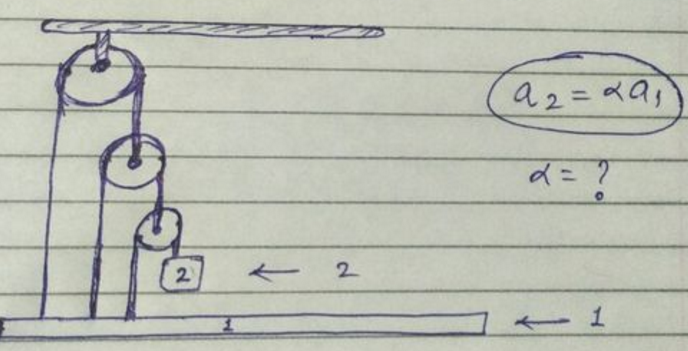# Constraint relation 01Using constraint equations, show that the relationship between $a_1$ and $a_2$ can be expressed as $a_2 = \alpha \times a_1$. Find $\alpha$.

Assume both the pulleys and string are light and friction is absent everywhere. Where $a_1$ and $a_2$ are vectors.

×